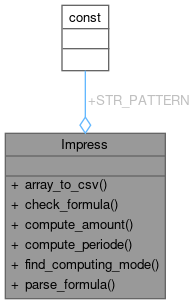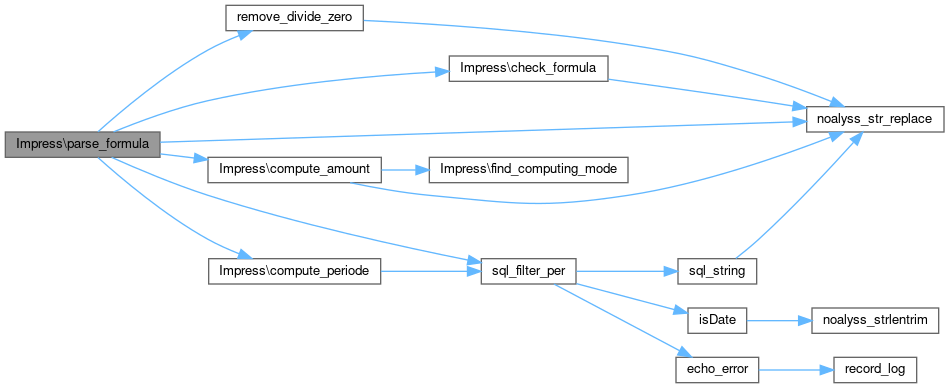noalyss  Version-7
Impress Class Reference
Collaboration diagram for Impress:Static Public Member Functions

with the handle of a successull query, echo each row into CSV and send it directly More...

static check_formula (\$p_string)
Check if formula doesn't contain php injection. More...

static parse_formula (\$p_cn, \$p_label, \$p_formula, \$p_start, \$p_end, \$p_eval=true, \$p_type_date=0, \$p_sql="")
Purpose Parse a formula. More...

Detailed Description

Definition at line 28 of file impress.class.php.

Member Function Documentation

 static Impress::array_to_csv ( \$array, \$aheader, \$p_filename )
static

with the handle of a successull query, echo each row into CSV and send it directly

Parameters
 type \$array of data type \$aheader double array, each item of the array contains a key type (num) and a key title

Definition at line 254 of file impress.class.php.

References \$array, \$e, \$row, and \$value.

Referenced by Anc_GrandLivre\display_csv().

255  {
256  \$file_csv=new Noalyss_Csv(\$p_filename);
258  {
260  }
261  \$file_csv->write();
262
263  // fetch all the rows
264  for (\$i=0; \$i<count(\$array); \$i++)
265  {
266  \$row=\$array[\$i];
267  \$e=0;
268  // for each rows, for each value
269  foreach (\$array[\$i] as \$key=> \$value)
270  {
272  continue;
273
275  {
277  {
278  case 'num':
280  break;
281  default:
283  }
284  }
285  else
286  {
288  }
289  \$e++;
290  }
291  \$file_csv->write();
292  }
293  }
\$value
Manage the CSV : manage files and write CSV record.
 static Impress::check_formula ( \$p_string )
static

Check if formula doesn't contain php injection.

Parameters
 string
Returns
true if the formula is good otherwise false

Definition at line 194 of file impress.class.php.

Referenced by parse_formula().

195  {
196  // the preg_match gets too complex if we want to add a test
197  // for parenthesis, math function...
198  // So I prefer remove them before testing
199  \$p_string=str_replace("round","",\$p_string);
200  \$p_string=str_replace("abs","",\$p_string);
201  \$p_string=str_replace("(","",\$p_string);
202  \$p_string=str_replace(")","",\$p_string);
203  // for the inline test like \$a=(cond)?value:other;
204  \$p_string=str_replace("?","+",\$p_string);
205  \$p_string=str_replace(":","+",\$p_string);
206  \$p_string=str_replace(">=","+",\$p_string);
207  \$p_string=str_replace("<=","+",\$p_string);
208  \$p_string=str_replace(">","+",\$p_string);
209  \$p_string=str_replace("<","+",\$p_string);
210  // eat Space + comma
211  \$p_string=str_replace(" ","",\$p_string);
212  \$p_string=str_replace(",","",\$p_string);
213  // Remove D/C/S
214  \$p_string=str_replace("c","",\$p_string);
215  \$p_string=str_replace("d","",\$p_string);
216  \$p_string=str_replace("s","",\$p_string);
217  // Remove T,t
218  \$p_string=str_replace("t","",\$p_string);
219
220  // remove date
221  \$p_string= preg_replace("/FROM*=*[0-9]+/", "", \$p_string);
222  // remove comment
223  \$p_string= preg_replace("/#.*/", "", \$p_string);
224  // remove \$C=
225  \$p_string= preg_replace('/\\$[a-z]*[A-Z]*[0-9]*[A-Z]*[a-z]*/', "", \$p_string);
226  \$p_string= preg_replace('/=/', "", \$p_string);
227
228  // remove account
229  \$p_string= preg_replace("/\[[0-9]*[A-Z]*%*\]/", "", \$p_string);
230
231  \$p_string= preg_replace("/\+|-|\/|\*/", "", \$p_string);
232  \$p_string= preg_replace("/[0-9]*\.*[0-9]/", "", \$p_string);
233
234  //********************************************************************************************************************
235  // If the string is empty then formula should be good
236  //
237  //********************************************************************************************************************
238  if (\$p_string == '')
239  {
240  return true;
241  }
242  else
243  {
244  return false;
245  }
246  }
 static Impress::parse_formula ( \$p_cn, \$p_label, \$p_formula, \$p_start, \$p_end, \$p_eval = `true`, \$p_type_date = `0`, \$p_sql = `""` )
static

Purpose Parse a formula.

Parameters
 \$p_cn connexion \$p_label \$p_formula \$p_eval true if we eval here otherwise the function returns a string which must be evaluated \$p_type_date : type of the date 0 for accountant period or 1 for calendar
Returns
array
Note
special value for the clause FROM=00.0000

Definition at line 43 of file impress.class.php.

References \$detail, \$e, \$from, \$g_user, \$last, \$line, \$oPeriode, \$p_end, \$p_start, \$periode, \$result, \$ret, \$year, check_formula(), Periode, sql_filter_per(), and trim().

Referenced by Acc_Bilan\compute_formula(), Anticipation\display(), and Acc_Report\get_row().

44  {
45  global \$g_user;
46  if ( Impress::check_formula(\$p_formula) == false)
47  {
48  if ( \$p_eval == true)
49  return array('desc'=>\$p_label.' Erreur Formule!',
50  'montant'=>0);
51  else
52  return \$p_formula;
53
54  }
55  if ( \$p_type_date == 0 )
56  \$cond=sql_filter_per(\$p_cn,\$p_start,\$p_end,'p_id','j_tech_per');
57  else
58  \$cond="( j_date >= to_date('\$p_start','DD.MM.YYYY') and j_date <= to_date('\$p_end','DD.MM.YYYY'))";
59
60  include_once("class/acc_account_ledger.class.php");
61
62  while (preg_match_all("(\[[0-9]*[A-Z]*%*c*d*s*\])",\$p_formula,\$e) == true)
63  {
64
65  // remove the [ ]
66  \$x=\$e;
67  foreach (\$x as \$line)
68  {
69  \$compute='all';
70  if ( strpos(\$line,'d') != 0 )
71  \$compute='deb';
72  if ( strpos(\$line,'c') != 0 )
73  \$compute='cred';
74  if ( strpos(\$line,'s') != 0 )
75  \$compute='signed';
76  \$line=str_replace ("[","",\$line);
77  \$line=str_replace ("]","",\$line);
78  \$line=str_replace ("d","",\$line);
79  \$line=str_replace ("c","",\$line);
80  \$line=str_replace ("s","",\$line);
81  // If there is a FROM clause we must recompute
82  // the time cond
83
84  if (\$p_type_date == 0 && preg_match ("/FROM=[0-9]+\.[0-9]+/", \$p_formula,\$afrom) == 1 )
85  {
86  // There is a FROM clause
87  // then we must modify the cond for the periode
88  \$from=str_replace("FROM=","",\$afrom);
89
90  // Get the periode
91  /*! \note special value for the clause FROM=00.0000
92  */
93  if ( \$from == '00.0000' )
94  {
95
96  // retrieve the first month of this periode
97  \$user_periode=\$g_user->get_periode();
98  \$oPeriode=new Periode(\$p_cn);
99  \$periode=\$oPeriode->get_exercice(\$user_periode);
100  list(\$first,\$last)=\$oPeriode->get_limit(\$periode);
101  \$ret=\$first->get_date_limit();
102  \$end_date=\$oPeriode->get_date_limit(\$p_end);
103  if (\$ret == null ) throw new Exception ('Pas de limite à cette période',1);
104  \$cond=sql_filter_per(\$p_cn,\$ret['p_start'],\$end_date['p_end'],'date','j_tech_per');
105
106
107  }
108  else
109  {
110  \$oPeriode=new Periode(\$p_cn);
111  try
112  {
113  \$pfrom=\$oPeriode->find_periode('01.'.\$from);
114  \$cond= sql_filter_per(\$p_cn, \$pfrom, \$p_end,'p_id','j_tech_per');
115  }
116  catch (Exception \$exp)
117  {
118  /* if none periode is found
119  then we take the first periode of the year
120  */
121  \$user_periode=\$g_user->get_periode();
122
123  \$year=\$oPeriode->get_exercice(\$user_periode);
124  list(\$first,\$last)=\$oPeriode->get_limit(\$year);
125  \$ret=\$first->get_date_limit();
126  \$end_date=\$oPeriode->get_date_limit(\$p_end);
127  if (\$ret == null ) throw new Exception ('Pas de limite à cette période',1);
128  \$cond=sql_filter_per(\$p_cn,\$ret['p_start'],\$end_date['p_end'],'date','j_tech_per');
129  }
130  }
131  }
132
133  if ( strpos(\$p_formula,"FROM") != 0)
134  {
135  // We remove FROM out of the p_formula
136  \$p_formula=substr_replace(\$p_formula,"",strpos(\$p_formula,"FROM"));
137  }
138
139  // Get sum of account
140  \$P=new Acc_Account_Ledger(\$p_cn,\$line);
141  \$detail=\$P->get_solde_detail(\$cond.\$p_sql);
142
143
144  if ( \$compute=='all')
145  \$i=\$detail['solde'];
146  if ( \$compute=='deb')
147  \$i=\$detail['debit'];
148  if ( \$compute=='cred')
149  \$i=\$detail['credit'];
150  if ( \$compute=='signed')
151  \$i=\$detail['debit']-\$detail['credit'];
152  \$p_formula=str_replace(\$x,\$i,\$p_formula);
153  }
154  }
155
156  // \$p_eval is true then we eval and returns result
157  if ( \$p_eval == true)
158  {
159  \$p_formula="\\$result=".\$p_formula.";";
160  eval("\$p_formula");
161
162  while (preg_match("/\[([0-9]+)([Tt]*)\]/",trim(\$p_label),\$e) == 1)
163  {
164  \$nom = "!!".\$e."!!";
165  if (Impress::check_formula(\$e))
166  {
167  \$nom = \$p_cn->get_value ( "SELECT pcm_lib AS acct_name FROM tmp_pcmn WHERE pcm_val::text LIKE \$1||'%' ORDER BY pcm_val ASC LIMIT 1",array(\$e));
168  if(\$nom)
169  {
170  if(\$e == 'T') \$nom = strtoupper(\$nom);
171  if(\$e == 't') \$nom = strtolower(\$nom);
172  }
173  }
174  \$p_label = str_replace(\$e, \$nom, \$p_label);
175  }
176
177  \$aret=array('desc'=>\$p_label,
178  'montant'=>\$result);
179  return \$aret;
180  }
181  else
182  {
183  // \$p_eval is false we returns only the string
184  return \$p_formula;
185  }
186  }
var Periode
Definition: scripts.js:3087
Manage the account from the table jrn, jrnx or tmp_pcmn.
\$p_end
static check_formula(\$p_string)
Check if formula doesn&#39;t contain php injection.
sql_filter_per(\$p_cn, \$p_from, \$p_to, \$p_form='p_id', \$p_field='jr_tech_per')
Create the condition to filter on the j_tech_per thanks a from and to date.
Definition: ac_common.php:623
global \$g_user
Find the default module or the first one.
Definition: action.inc.php:24
function trim(s)